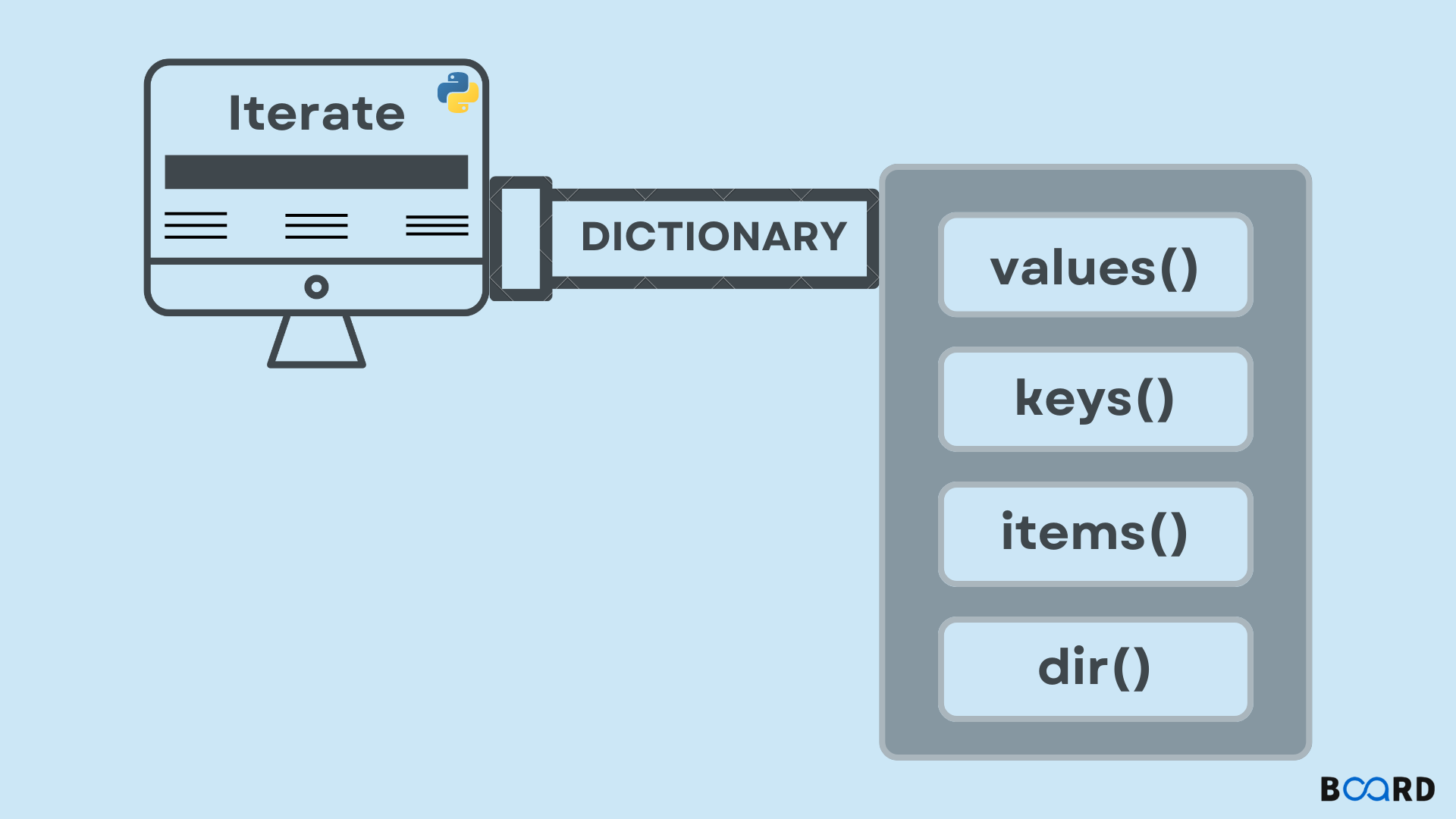Dictionary Python

# Iterate over a dictionary in Python### Employing the keys() Method

Python dictionaries include a convenient method called keys that makes it simple to loop through every initialized key in a dictionary ().

Remember that since Python 3, this function returns a view object rather than a list. Like its name implies, a view object is a representation of some data.

This means that while iterating through the data won't be a problem, storing the list of keys requires materialization. Which is simply accomplished by sending the view object provided to a list function Object().

Example :

 my_dict = {'alpha': 5, 'beta': 4, 'gamma': 3}# Here we're just 'looking' at the keys,# we're not actually constructing a list # out of themkey_view = my_dict.keys()print("Key view:", key_view)print("Type:", type(key_view),end="\n\n")# Here we're materializing the keys# into a list ofo keyskey_list = list(my_dict.keys())print("Key list:", key_list)print("Type: ", type(key_list),end="\n\n")# And as we can see, view can be easily be# used for iterating over a dictionaryfor key in my_dict.keys():    print(key, ':', my_dict[key])

Output:

 Key view: dict_keys(['alpha', 'beta', 'gamma'])Type: Key list: ['alpha', 'beta', 'gamma']Type:  alpha : 5beta : 4gamma : 3

Another approach might be:

 my_dict = {'alpha': 5, 'beta': 4, 'gamma': 3}for key in my_dict:    print(key, ':', my_dict[key])
write your code here: Coding Playground

When a dictionary is used in conjunction with the in keyword, the dictionary calls its __iter__() method. The iterator that this method returns is then used to implicitly iterate across the dictionary's keys.

## Making use of the values() Method

The values() method returns a view object just like the keys() method does, but it iterates through values rather than keys :

my_dict = {'alpha': 5, 'beta': 4, 'gamma': 3}

# Inspecting the view of the values
# in the dictionary
key_list = list(my_dict.values())
print("Value list:", key_list)
print("Type: ", type(key_list), end="\n\n")

for value in my_dict.values():
print(value, end=" ")

Output:

 Value list: [5, 4, 3]Type:  5 4 3
write your code here: Coding Playground

This approach merely returns values, as opposed to the prior one. When you are not worried about keys, it is helpful.

## The items() method is used

The items() method returns a view object, similarly like the keys() and values() methods, but instead of only iterating through keys or values, iterates through (key,value) pairs.

Let's examine how everything functions:

my_dict = {'alpha': 5, 'beta': 4, 'gamma': 3}

# Inspecting the view of the (key,value) pairs
key_list = list(my_dict.items())
print("(key,value) pair list:", key_list)
print("Type: ", type(key_list), end="\n\n")

for item in my_dict.items():
print(item, end=" ")

Output:

 (key,value) pair list: [('alpha', 5), ('beta', 4), ('gamma', 3)]Type:  ('alpha', 5) ('beta', 4) ('gamma', 3)

We may utilize tuple unpacking and extract both keys and values simultaneously by utilizing variables for each value in a tuple:

 for key, value in my_dict.items():    print(key,':',value)
write your code here: Coding Playground

It is crucial to remember that in earlier versions of Python 2, the functions items(), keys(), and values() all returned copies of dictionary data. However, they return a view object in Python 3.

These are more efficient since they offer a dynamic view, and they also instantly update the view object if any changes are made to the original dictionary (and vice versa).